Question

# The following graph shows the aggregate demand (AD) curve in a hypothetical economy. At point A,...

The following graph shows the aggregate demand (AD) curve in a hypothetical economy. At point A, the price level is 120, and the quantity of output demanded is \$500 billion. Moving up along the aggregate demand curve from point A to point B, the price level rises to 140, and the quantity of output demanded falls to \$300 billion.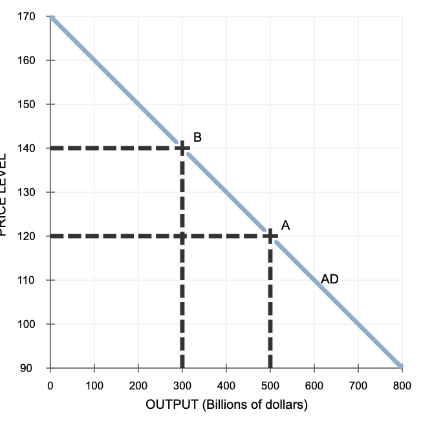As the price level rises, the purchasing power of households' real wealth will _______  causing the quantity of output demanded to _______ .This phenomenon is known as the _______ effect.

Additionally, as the price level rises, the impact on the domestic interest rate will cause the real value of the dollar to _______ in foreign exchange markets. The number of domestic products purchased by foreigners (exports) will therefore _______ , and the number of foreign products purchased by domestic consumers and firms (imports) will _______ .Net exports will therefore _______ , causing the quantity of domestic output demanded to _______ . This phenomenon is known as the _______ effect.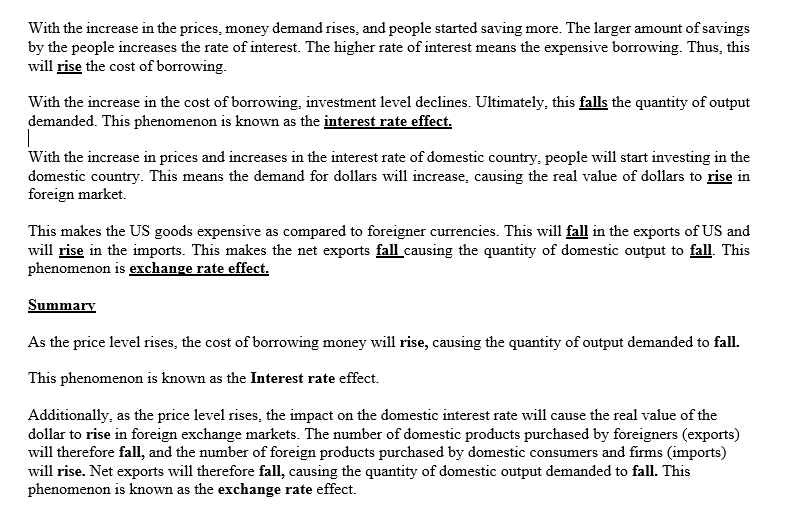As price level rises, purchasing power of household real wealth will decrease, causing the quantity of output demanded to decreases. This phenomenon is known as the income/real effect

As price level rises, real value of dollar to appreciates  in foreign exchange market.

Export will fall and import will rise  Net export will therefore decreases causing the domestic output demand to decrease. This phenomenon is known as the substitution effect.

Aggregate demand is negatively sloped meaning increase in price leads to decrease in quantity demanded. Interest rate effect and exchange rate effect are the two reasons explaining the slope of aggregate demand.

Blanks :

Decrease, decrease, wealth

Rise, decrease, increase, decrease, decrease, exchange rate

When the domestic price level falls, foreign goods become relatively more expensive than domestic goods, and domestic goods become relatively less expensive than foreign imported goods. Exports of domestic goods to foreigners therefore rise, while domestic imports of foreign goods fall. Net exports (exports minus imports) therefore rise, leading to a rise in the quantity of domestic output demanded.

The effect of a change in the domestic price level on the quantity of real GDP demanded via its effects on net exports is known as the open economy effect (sometimes referred to as the exchange rate effect).

source: cengage
[REMOVED]

As the price level rises, the purchasing power of households' real wealth willfall   , causing the quantity of output demanded tofall   . This phenomenon is known as thewealth   effect.

Additionally, as the price level rises, the impact on the domestic interest rate will cause the real value of the dollar torise   in foreign exchange markets. The number of domestic products purchased by foreigners (exports) will thereforefall   , and the number of foreign products purchased by domestic consumers and firms (imports) willrise   . Net exports will thereforefall   , causing the quantity of domestic output demanded tofall   . This phenomenon is known as theexchange rate   effect.

source: MINDAP

#### Earn Coins

Coins can be redeemed for fabulous gifts.

Similar Homework Help Questions
• ### The following graph shows the aggregate demand curve in a hypothetical economy. Assume that the economy's money supply remains fixed.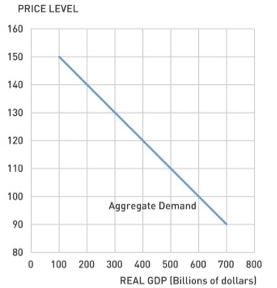Aggregate demand curve The following graph shows the aggregate demand curve in a hypothetical economy. Assume that the economy's money supply remains fixed. Which of the following are reasons the aggregate demand curve is downward sloping? Check all that apply. A lower price level leads to a lower interest rate. A higher price level makes domestically produced goods more expensive than foreign goods. A lower price level increases consumption through the income effect. As the aggregate price level rises, the purchasing power of households' saving balances...

• ### Which would most likely shift the aggregate supply curve? A change in the prices of _____....

Which would most likely shift the aggregate supply curve? A change in the prices of _____. domestic products foreign products financial assets resources A decrease in aggregate demand in the short run will reduce _____. both real output and the price level the price level and increase the real domestic output the real domestic output and have no effect on the price level the price level and have no effect on real domestic output The economy's long-run AS curve assumes...

• ### The following graph shows an increase in aggregate demand (AD) in a hypothetical country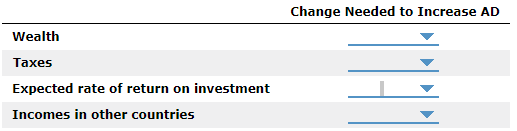The following graph shows an increase in aggregate demand (AD) in a hypothetical country. Specifically, aggregate demand shifts to the right from AD1 to AD2, causing the quantity of output demanded to rise at all price levels. For example, at a price level of 140, output is now \$400 billion, where previously it was \$300 billion. The following table lists several determinants of aggregate demand. Complete the table by indicating the change in each determinant necessary to increase aggregate demand.

• ### The following graph shows a decrease in aggregate demand (AD) in a hypothetical country. Specifically, aggregate demand shifts to the left from AD1 to AD2, causing the quantity of output demanded to fall at all price levels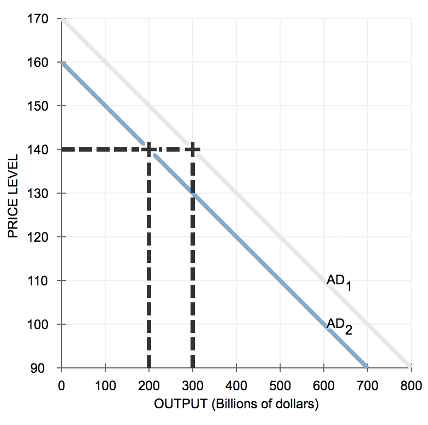4. Determinants of aggregate demand The following graph shows a decrease in aggregate demand (AD) in a hypothetical country. Specifically, aggregate demand shifts to the left from AD1 to AD2, causing the quantity of output demanded to fall at all price levels For example, at a price level of 140, output is now \$200 billion, where previously it was \$300 billion. The following table lists several determinants of aggregate demand. Complete the table by indicating the change in each determinant necessary to decrease...

• ### The following graph shows the short-run aggregate supply curve (AS), the aggregate demand curve (AD), and the long-run aggregate supply curve (LRAS) for a hypothetical economy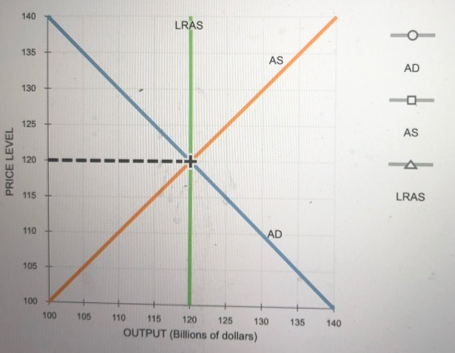9. Economic fluctuations II The following graph shows the short-run aggregate supply curve (AS), the aggregate demand curve (AD), and the long-run aggregate supply curve (LRAS) for a hypothetical economy. Initially, the expected price level is equal to the actual price level, and the economy is in long-run equilibrium at its natural level of output, \$120 billion. Suppose a bout of severe weather drives up agricultural costs, increases the costs of transporting goods and services, and increases the costs of producing goods...

• ### The aggregate-demand curve O shows an inverse relation between the price level and the quantity of...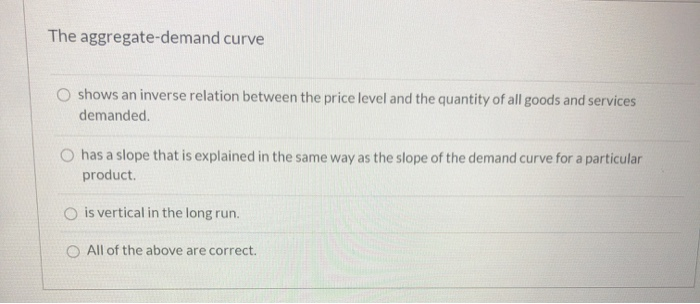The aggregate-demand curve O shows an inverse relation between the price level and the quantity of all goods and services demanded. O has a slope that is explained in the same way as the slope of the demand curve for a particular product O is vertical in the long run. O All of the above are correct. Question 24 If aggregate demand shifts left, then in the short run the price level and real GDP both rise. O the price...

• ### Economics chart The following graph shows the economy in long-run equilibrium at the price level of...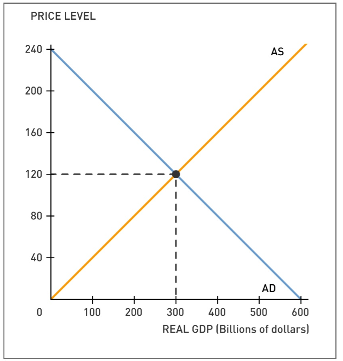Economics chart The following graph shows the economy in long-run equilibrium at the price level of 120 and potential output of \$300 billion. Suppose several foreign economies experience severe recessions, causing foreign purchases of domestic goods and services to decline sharply. Shift the short-run aggregate supply (AS) curve or the aggregate demand (AD) curve to show the short-run impact of the economic turmoil abroad. Tool tip: Click and drag one or both of the curves. Curves will snap into position, so if...

• ### The following table shows the initial level of aggregate demand (AD) and te supply (AS) for the economy of Adanac.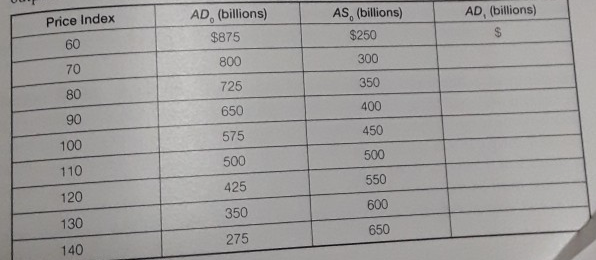The following table shows the initial level of aggregate demand (AD) and te supply (AS) for the economy of Adanac. The full-employment level of output is \$500 billion.  a. Draw the corresponding initial aggregate demand and aggregate supply curve (AD0 and AS0). b. What is the initial equilibrium price level and level of real GDP?  c. At this initial equilibrium (AD0 and AS0), is Adanac experiencing either a recessionary or inflationary gap? If so, how large a gap exists? d. Suppose the aggregate demand in...

• ### 26. are The four components of planned aggregate expenditures a. Consumption, investment, inventories, and government purchases....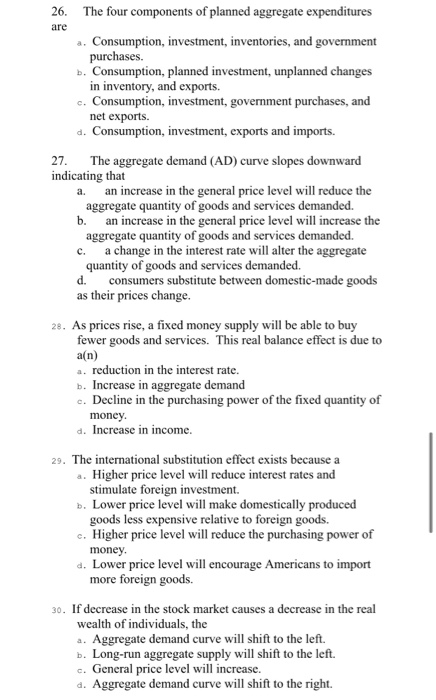26. are The four components of planned aggregate expenditures a. Consumption, investment, inventories, and government purchases. b. Consumption, planned investment, unplanned changes in inventory, and exports. c. Consumption, investment, government purchases, and net exports. d. Consumption, investment, exports and imports. 27. The aggregate demand (AD) curve slopes downward indicating that a. an increase in the general price level will reduce the aggregate quantity of goods and services demanded. b. an increase in the general price level will increase the aggregate...

• ### Question 21 1 pts Use the following table which shows the aggregate demand and aggregate supply...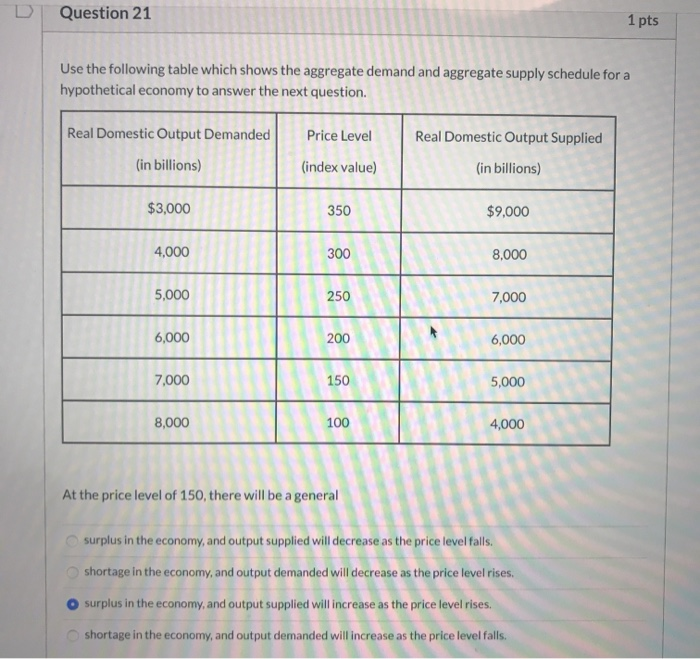Question 21 1 pts Use the following table which shows the aggregate demand and aggregate supply schedule for a hypothetical economy to answer the next question. Real Domestic Output Demanded Price Level Real Domestic Output Supplied (in billions) (index value) (in billions) \$3,000 350 \$9,000 4,000 300 8,000 5,000 250 7,000 6,000 200 6,000 7,000 150 5,000 8,000 100 4,000 At the price level of 150, there will be a general surplus in the economy, and output supplied will decrease...